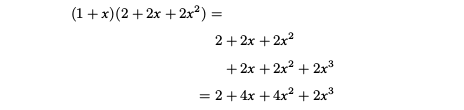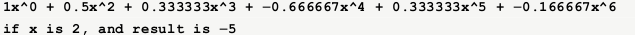在数值计算中，有时我们需要对多项式进行相加或相乘，此外，还经常需要对多项式进行求值。例如，假定我们需要对两个多项式进行乘法运算：
$$(1+x+\frac{x^2}{2}-\frac{x^3}{6}) (1+x+x^2+x^3)= 1 + \frac{x^2}{2} + \frac{x^3}{3} - \frac{2x^4}{3} + \frac{x^5}{3} - \frac{x^6}{3}$$

## 多项式的表示

我们可以定义一个Poly类用来表示多项式，在Poly中，我们使用数组来存储多项式的系数，由于系数作为数组的元素，那么系数对应的下标就是多项式的幂，例如，假定我们使用Poly来表示多项式$5+10x+8x^2+20x^4$，那么数组的结构就如下图所示：我们可以用Poly来表示多项式，例如，我们可以用Poly<int>(2, 2)来表示$2x^2$，当然，我们可以通过全局函数plus()来组合多项式，例如：

那么，可以看到，poly就可以用来表示$0.5x^2 + 9.9x^4$，这时，若需要对多项式进行求值，例如，令$x=2$，那么可以使用Poly的成员函数evaluate()来进行求值：

下面给出Poly的具体实现：

## 多项式的加法

在对多项式进行加法运算时，我们需要对幂相同的项系数进行累加：plus()函数实现多项式的加法：

## 多项式的乘法

在对多项式进行乘法运算时，我们可以使用乘法分配率：# 多项式的求值

要是我们知道变量的值，那就可以对多项式进行求值了。例如可以直接对多项式进行求值：
$$a_4x^4+a_3x^3+a_2x^2+a_1x+a_0$$
可以发现，假定多项式的最高次幂是N，那么直接对多项式进行求值，计算复杂度是$O(N^2)$，然而，我们可以使用Horner算法，将计算复杂度降至$O(N)$，例如：
$$a_4x^4+a_3x^3+a_2x^2+a_1x+a_0 = (((a_4x+a_3)x + a_2)x+a_1)x+a_0$$
这样，对多项式进行求值只需要线性时间。

我们可以编写一个测试程序，验证以下多项式乘法：
$$(1+x+\frac{x^2}{2}-\frac{x^3}{6})(1+x+x^2+x^3) = 1 + \frac{x^2}{2} + \frac{x^3}{3} - \frac{2x^4}{3} + \frac{x^5}{3} - \frac{x^6}{6}$$

程序的运行输出：## 参考资料

Algorithms in C++ 3rd Edition by Robert Sedgewick